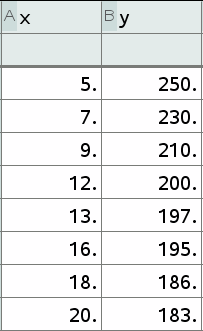# 2650 Tinspire App Quiz

37 Questions | Attempts: 166SettingsUsing the document Bivariate Data App Pages as a reference, answer the following questions. Dataset 135 is used for some questions - which you can load through the App, but a sample is also below.

• 1.
When you are given a data set in a SAC, which app should you use put it into your calculator
• 2.
Immediately after you have put your data into your calculator, which App do you need to run to refresh the values on the other pages?
• 3.
Which App should I run in order to populate the pages relating to Transformations?
• 4.
It is always good practice to check the values you have entered. On which page could I expect to check the values of the data in a table format?
• 5.
I need to look at the scatter plot of the bivariate data to determine which quadrant it might fit into. Which page would I go to?
• 6.
I want a full list of details relating to the Linear Regression equation, form and strength, correlation coefficient and coefficient of deterimation. Which page will offer me a summary of these details?
• 7.
The only way to truly tell if a set of data is linear is to look at its residual plot. Which page would I find the residual plot for the least squares regression line?
• 8.
I have just run the transformation App to find the best transformation for the data set. Which page would give the equation for the line for the best transformation?
• 9.
While a transformation may be determined to be the 'best' transformation because of its coefficient of determination, thats not enough to prove it will be accurate. You still need to look at the residual plot to see that the data is linear AFTER it has been transformed. Where would I find the residual plot for an x squared transformation?
• 10.
While a transformation may be determined to be the 'best' transformation because of its coefficient of determination, thats not enough to prove it will be accurate. You still need to look at the residual plot to see that the data is linear AFTER it has been transformed. Where would I find the residual plot for a y squared transformation?
• 11.
While a transformation may be determined to be the 'best' transformation because of its coefficient of determination, thats not enough to prove it will be accurate. You still need to look at the residual plot to see that the data is linear AFTER it has been transformed. Where would I find the residual plot for a log(x) transformation?
• 12.
While a transformation may be determined to be the 'best' transformation because of its coefficient of determination, thats not enough to prove it will be accurate. You still need to look at the residual plot to see that the data is linear AFTER it has been transformed. Where would I find the residual plot for a log(y) transformation?
• 13.
While a transformation may be determined to be the 'best' transformation because of its coefficient of determination, thats not enough to prove it will be accurate. You still need to look at the residual plot to see that the data is linear AFTER it has been transformed. Where would I find the residual plot for a 1/x transformation?
• 14.
While a transformation may be determined to be the 'best' transformation because of its coefficient of determination, thats not enough to prove it will be accurate. You still need to look at the residual plot to see that the data is linear AFTER it has been transformed. Where would I find the residual plot for a 1/y transformation?
• 15.
Sometimes we are asked for the equation for a transformation that is NOT the best transformation. Which page will offer me a summary of all the values for a & b transformations in order to create an equation from quadrant 1 transformations?
• 16.
Sometimes we are asked for the equation for a transformation that is NOT the best transformation. Which page will offer me a summary of all the values for a & b transformations in order to create an equation from quadrant 2 transformations?
• 17.
Sometimes we are asked for the equation for a transformation that is NOT the best transformation. Which page will offer me a summary of all the values for a & b transformations in order to create an equation from quadrant 3 transformations?
• 18.
Sometimes we are asked for the equation for a transformation that is NOT the best transformation. Which page will offer me a summary of all the values for a & b transformations in order to create an equation from quadrant 4 transformations?
• 19.
I have a table of values in an exercise from the book. It is asking me to populate the table with log, squared and reciprocal values. Which App will help me to find all the values in one hit?
• 20.
If x is 70, what is log(x)? (2 decimal places)
• 21.
If x is 22, what is the reciprocal of x? (3 decimal places)
• 22.
If x is 15, what is the square of x?
• 23.
If y is 10, what is the reciprocal of y? (1 decimal place)
• 24.
If y is 30, what is the log of y? (2 decimal places)
• 25.
If y is 22, what is y squared?Back to top